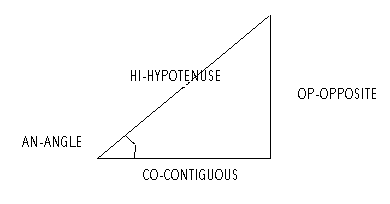<

Trigonometric Relations

Only read this chapter when you have trained the Calculated values chapter with many examples.You can use several trigonometric functions when you define calculated values.

For instance, if you want the length of the hypotenuse to be calculated from the opposite segment and the angle, then you can use AN_OP_HI(). So if for instance the opposite length is [1:3L] and the angle is [1:1A], then you must type the following expression in the calculated field of the hypotenuse length:

AN_OP_HI( [1:1A] , [1:3L] )

If you want the angle to be calculated from the hypotenuse and the contiguous segment, then you can use HI_CO_AN(). So if for instance the hypotenuse length is [1:2L] and the contiguous length is [1:3L], Audemars Piguet Replica then you must type the following expression in the calculated field of the angle:

HI_CO_AN( [1:2L] , [1:3L] )

Here are the trigonometric functions available:

HI_OP_AN( HI , OP ) = AN

HI_CO_AN( HI , CO ) = AN

CO_OP_AN( CO, OP ) = AN

AN_OP_HI( AN , OP ) = HI

AN_CO_HI( AN , CO ) = HI

CO_OP_HI( CO , OP ) = HI

HI_CO_OP( HI , CO ) = OP

HI_AN_OP( HI , AN ) = OP

AN_CO_OP( AN , CO ) = OP

HI_OP_CO( HI , OP ) = CO

HI_AN_CO( HI , AN ) = CO

AN_OP_CO( AN , OP ) = CO

Please, be careful to type the parameters in the right sequence. For instance, if you want to calculate the contiguous segment from opposite segment and angle, you can't use OP_AN_CO because this function doesn't exist. You must choose one from the list above: AN_OP_CO, so the first parameter must be the angle and the second the opposite segment.

To study this chapter you should first have a look at the models with trigonometric relations which are already created, and once you have seen many of them try creating your own models. With each already created model you want to examine, follow these steps:

• Test the model by using it when you are entering an order. Watch how it works, write down which value(s) are calculated by trigonometric relations.
• Choose General->Models->Add / Change / Delete.
• Type the model code and press OK.
• Press the space bar until "VALUE" is included in the window caption.
• Select the calculated value. You know how to do that from chapter Members and values.
• Choose Drawing->Object properties or press Enter. You see a window with the properties of the value.
• You will find in this window that in all the already created models NoEdit has been chosen for calculated values, for simplicity reasons.
• Watch the expression in Calculated field. Here you can see what function is used and what symbols are its parameters. If you don't know what values correspond to those symbols, write the expression down. Once you press Cancel you can know the symbol of each value by selecting it with the mouse while you are in the VALUE setting.
• Press Cancel.
• Choose File->Exit.
• Answer No to the question: ? Do you want to save the information ?